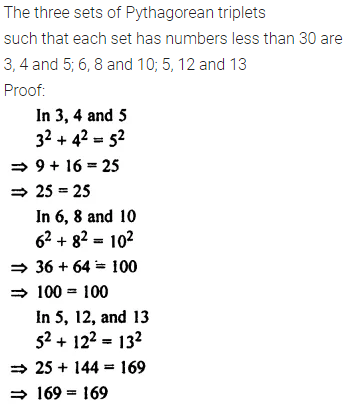# Selina Concise Mathematics Class 8 ICSE Solutions Chapter 3 Squares and Square Roots

## Selina Concise Mathematics Class 8 ICSE Solutions Chapter 3 Squares and Square Roots

Selina Publishers Concise Mathematics Class 8 ICSE Solutions Chapter 3 Squares and Square Roots

### Squares and Square Roots Exercise 3A – Selina Concise Mathematics Class 8 ICSE Solutions

Question 1.
Find the square of :
(i) 59
(ii) 63
(iii) 15
Solution:Question 2.
By splitting into prime factors, find the square root of :
(i) 11025
(ii) 396900
(iii) 194481
Solution: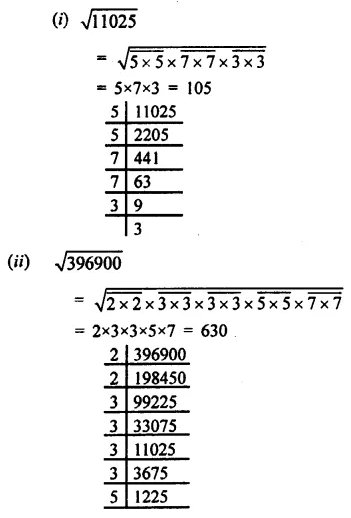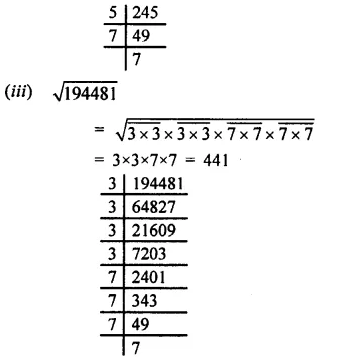Question 3.
(i) Find the smallest number by which 2592 be multiplied so that the product is a perfect square.
(ii) Find the smallest number by which 12748 be multiplied so that the product is a perfect square?
Solution: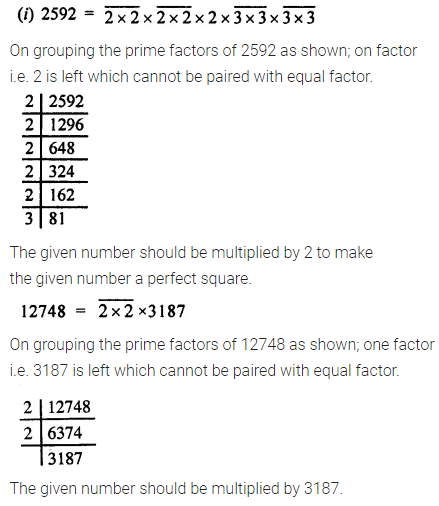Question 4.
Find the smallest number by which 10368 be divided, so that the result is a perfect square. Also, find the square root of the resulting numbers.
Solution: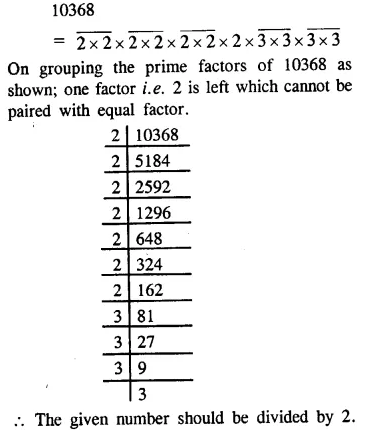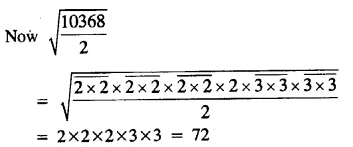Question 5.
Find the square root of :
(i) 0.1764
(ii) $$96\frac { 1 }{ 25 }$$
(iii) 0.0169
Solution: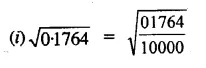Question 6.
Evaluate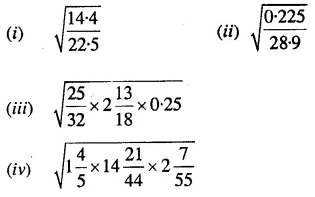Solution: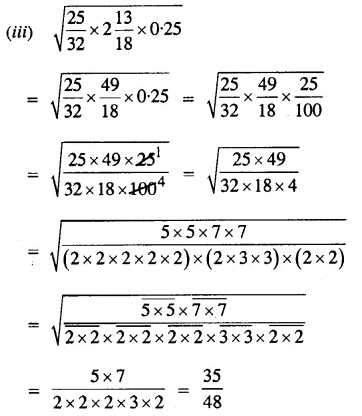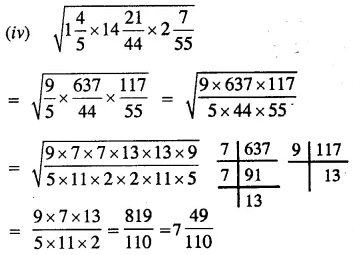Question 7.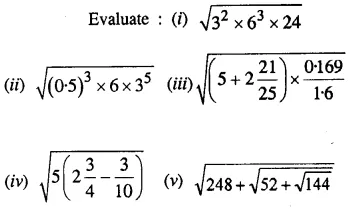Solution: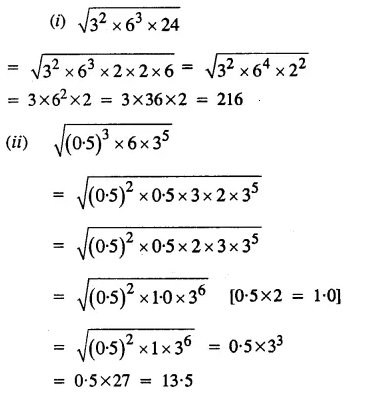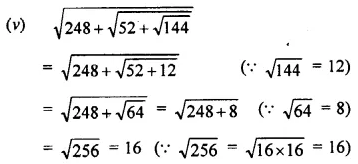Question 8.
A man, after a tour, finds that he had spent every day as many rupees as the number of days he had been on tour. How long did his tour last, if he had spent in all ₹ 1,296
Solution: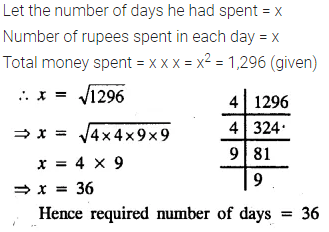Question 9.
Out of 745 students, maximum are to be arranged in the school field for a P.T. display, such that the number of rows is equal to the number of columns. Find the number of rows if 16 students were left out after the arrangement.
Solution:Question 10.
13 and 31 is a strange pair of numbers such that their squares 169 and 961 are also mirror images of each other. Find two more such pairs.
Solution:Question 11.
Find the smallest perfect square divisible by 3, 4, 5 and 6.
Solution:Question 12.
If √784 = 28, find the value of:
(i) √7.84 + √78400
(ii) √0.0784 + √0.000784
Solution:### Squares and Square Roots Exercise 3B – Selina Concise Mathematics Class 8 ICSE Solutions

Question 1.
Find the square root of:
(i) 4761
(ii) 7744
(iii) 15129
(iv) 0.2916
(v) 0.001225
(vi) 0.023104
(vii) 27.3529
Solution: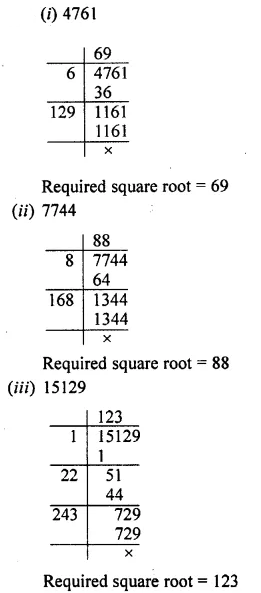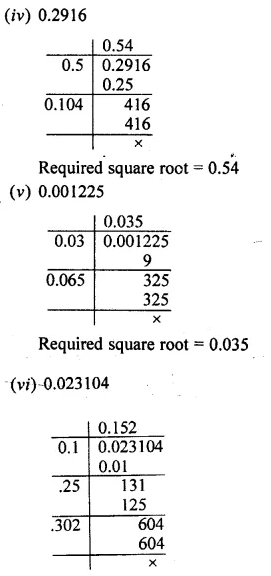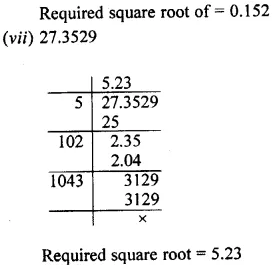Question 2.
Find the square root of:
(i) 4.2025
(ii) 531.7636
(iii) 0.007225
Solution: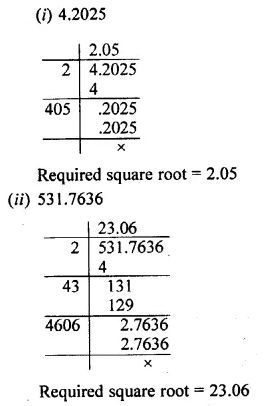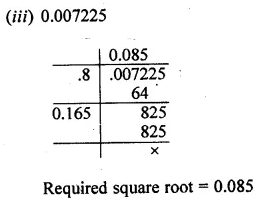Question 3.
Find the square root of:
(i) 245 correct to two places of decimal.
(ii) 496 correct to three places of decimal.
(iii) 82.6 correct to two places of decimal.
(iv) 0.065 correct to three places of decimal.
(v) 5.2005 correct to two places of decimal.
(vi) 0.602 correct to two places of decimal
Solution: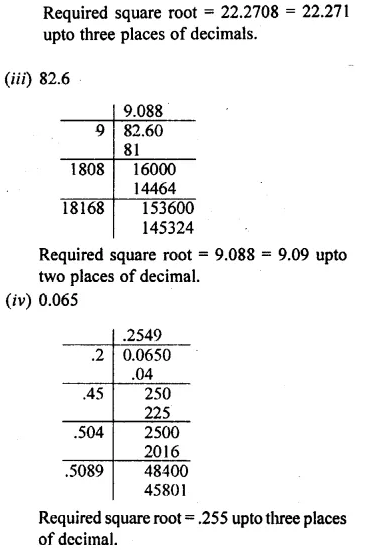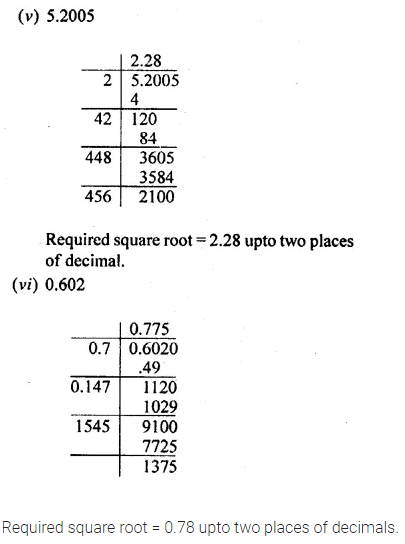Question 4.
Find the square root of each of the following correct to two decimal places:
(i) $$3\frac { 4 }{ 5 }$$
(ii) $$6\frac { 7 }{ 8 }$$
Solution:Question 5.
For each of the following, find the least number that must be subtracted so that the resulting number is a perfect square.
(i) 796
(ii) 1886
(iii) 23497
Solution: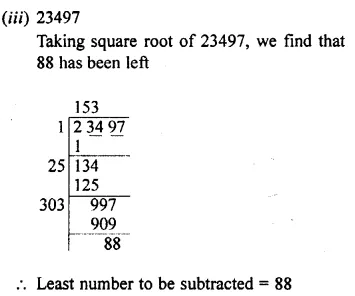Question 6.
For each of the following, find the least number that must be added so that the resulting number is a perfect square.
(i) 511
(ii) 7172
(iii) 55078
Solution: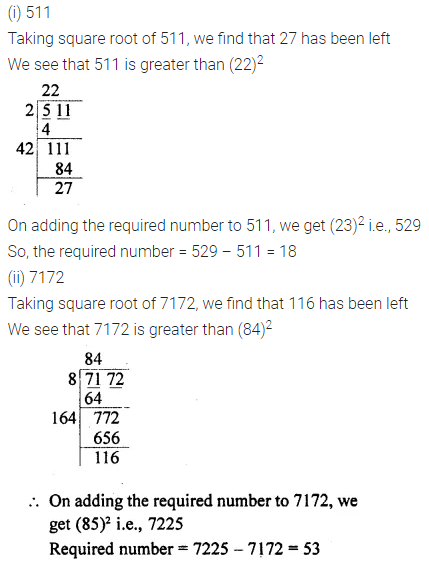Question 7.
Find the square root of 7 correct to two decimal places; then use it to find the value of $$\sqrt { \frac { 4+\sqrt { 7 } }{ 4-\sqrt { 7 } } }$$ correct to three significant digits.
Solution:Question 8.
Find the value of √5 correct to 2 decimal places; then use it to find the square root of $$\sqrt { \frac { 3-\sqrt { 5 } }{ 3+\sqrt { 5 } } }$$ correct to 2 significant digits.
Solution: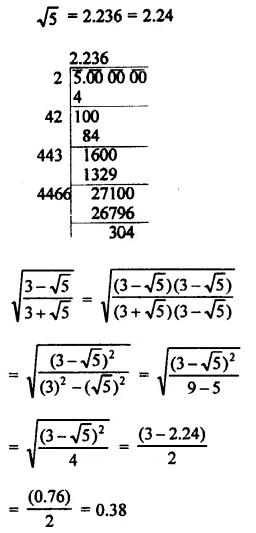Question 9.
Find the square root of:
(i) $$\frac { 1764 }{ 2809 }$$
(ii) $$\frac { 507 }{ 4107 }$$
(iii) $$\sqrt { 108\times 2028 }$$
(iv) 0.01 + √0.0064
Solution: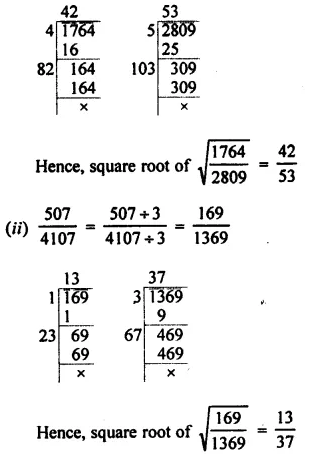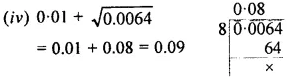Question 10.
Find the square root of 7.832 correct to :
(i) 2 decimal places
(ii) 2 significant digits.
Solution:Question 11.
Find the least number which must be subtracted from 1205 so that the resulting number is a perfect square.
Solution:Question 12.
Find the least number which must be added to 1205 so that the resulting number is a perfect square.
Solution: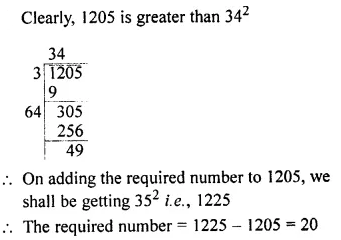Question 13.
Find the least number which must be subtracted from 2037 so that the resulting number is a perfect square.
Solution: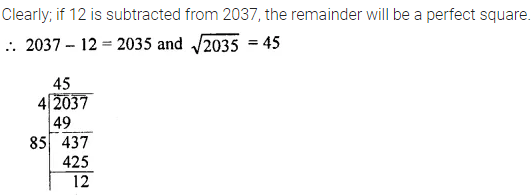Question 14.
Find the least number which must be added to 5483 so that the resulting number is a perfect square.
Solution:### Squares and Square Roots Exercise 3C – Selina Concise Mathematics Class 8 ICSE Solutions

Question 1.
Seeing the value of the digit at unit’s place, state which of the following can be square of a number :
(i) 3051
(ii) 2332
(iii) 5684
(iv) 6908
(v) 50699
Solution: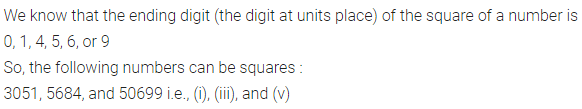Question 2.
Squares of which of the following numbers will have 1 (one) at their unit’s place :
(i) 57
(ii) 81
(iii) 139
(iv) 73
(v) 64
Solution: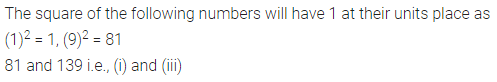Question 3.
Which of the following numbers will not have 1 (one) at their unit’s place :
(i) 322
(ii) 572
(iii) 692
(iv) 3212
(v) 2652
Solution:Question 4.
Square of which of the following numbers will not have 6 at their unit’s place :
(i) 35
(ii) 23
(iii) 64
(iv) 76
(v) 98
Solution:Question 5.
Which of the following numbers will have 6 at their unit’s place :
(i) 262
(ii) 492
(iii) 342
(iv) 432
(v) 2442
Solution: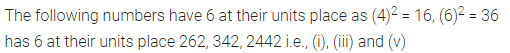Question 6.
If a number ends with 3 zeroes, how many zeroes will its square have?
Solution: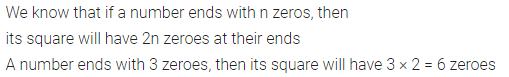Question 7.
If the square of a number ends with 10 zeroes, how many zeroes will the number have?
Solution:Question 8.
Is it possible for the square of a number to end with 5 zeroes ? Give reason.
Solution: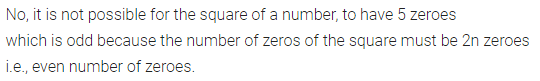Question 9.
Give reason to show that none of the numbers, given below, is a perfect square.
(i) 2162
(ii) 6843
(iii) 9637
(iv) 6598
Solution: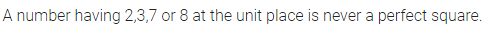Question 10.
State, whether the square of the following numbers is even or odd?
(i) 23
(ii) 54
(iii) 76
(iv) 75
Solution: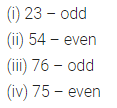Question 11.
Give reason to show that none of the numbers 640, 81000 and 3600000 is a perfect square.
Solution:Question 12.
Evaluate:
(i) 372 – 362
(ii) 852 – 842
(iii) 1012 – 1002
Solution: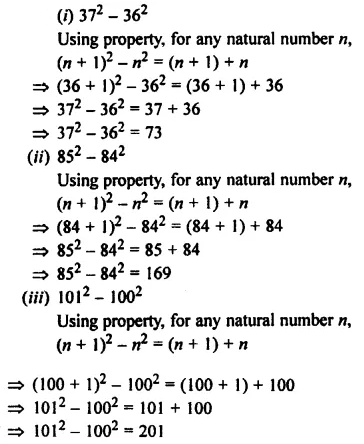Question 13.
Without doing the actual addition, find the sum of:
(i) 1 + 3 + 5 + 7 + 9 + 11 + 13 + 15 + 17 + 19 + 21 + 23
(ii) 1 + 3 + 5 + 7 + 9 + ……………… + 39 + 41
(iii) 1 + 3 + 5 + 7 + 9 + ………………… + 51 + 53
Solution:Question 14.
Write three sets of Pythagorean triplets such that each set has numbers less than 30.
Solution: